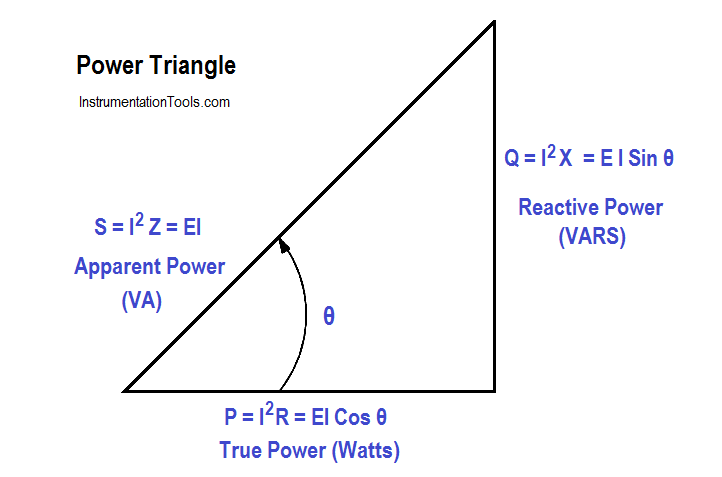# Power Triangle

In AC circuits, current and voltage are normally out of phase and, as a result, not all the power produced by the generator can be used to accomplish work. By the same token, power cannot be calculated in AC circuits in the same manner as in DC circuits.

The power triangle, shown in Figure 1, equates AC power to DC power by showing the relationship between generator output (apparent power – S) in volt-amperes (VA), usable power (true power – P) in watts, and wasted or stored power (reactive power – Q) in volt-amperes-reactive (VAR).

The phase angle (θ) represents the inefficiency of the AC circuit and corresponds to the total reactive impedance (Z) to the current flow in the circuit.Figure 1 : Power Triangle

The power triangle represents comparable values that can be used directly to find the efficiency level of generated power to usable power, which is expressed as the power factor (discussed later). Apparent power, reactive power, and true power can be calculated by using the DC equivalent (RMS value) of the AC voltage and current components along with the power factor.